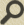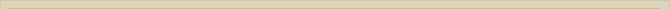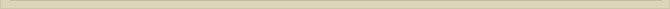## Fundamental Physics Experiment

When you are interested in physics you must read “Unbelievable“!

Quantum Mechanics (QM) assumes that electromagnetic fields are conservative. Theoretically it has been proved that this assumption, a basic premise, is false.

It is amazing that QM, claiming to be a theoretical science, ignores theoretical evidence!

(The Equivalence of Magnetic and Kinetic Energy section 4 The Electromagnetic Mass).

Scientists claim that QM is the most successful theory ever and therefore must be correct!

Should not a theoretical science be consistent with it’s premises?

QM ignores this and demands experimental proof.

The theoretical basis for QM is the Electromagnetic Theory (EM). The EM-theory assumes that magnetic, electric and electromagnetic fields own physical properties qualifying them to be (mathematical) vector fields.

The assumed vector properties of the EM-fields are of practical value because they allow vector calculations. As long as these calculations are consistent with the physical reality there is no objection. But how do we know for sure that these mathematical vector properties are valid whatever the circumstances?

This we do not know.

The EM-theory states that two parallel and similarly moving electric currents attract each other, while two parallel but opposite currents repel. This is empirically stated and therefore undeniable. However the EM-theory also claims that because of the assumed vector qualities, two parallel currents of particles with opposite sign must repel where the particles move in the same direction.

Do we know that for sure?

Science never empirically stated that an electron beam and a parallel proton beam, where the particles move in the same direction, repel. The fact that two electron currents behave vector-like as the EM-theory describes, does not necessarily imply that the same vector calculations are valid for the electron/proton beam.

Theoretical evidence disqualifying QM is ignored science. As a consequence this leaves only empirical evidence to falsify QM. In the ether theory ( www.paradox-paradigm.nl ) charged parallel currents, with particles moving in the same direction, always attract each other; irrespective of the charge of the particles.

(Read the explanation Magnetic attraction parallel currents with ether and/or the non-arguments of Nobel laureate Prof. ‘t Hooft)

The scientific conclusions of Theoretical Physics are more fantastic than any known fairytale!

Should not Quantum Mechanics be properly verified way of the Scientific Method?

The mentioned experiment is relatively simple. This experiment will demonstrate whether the EM-fields are real (mathematical) vector fields as is assumed.

Scientists, especially experimental physicists, should pursue the integrity of their profession despite the possible consequences.